1581134520

# PyTorch Tutorial - Convolutional Neural Network (CNN)

Learn all the basics you need to get started with this deep learning framework! In this part we will implement our first convolutional neural network (CNN) that can do image classification based on the famous CIFAR-10 dataset.

We will learn:

• Architecture of CNNs
• Convolutional Filter
• Max Pooling
• Determine the correct layer size
• Implement the CNN architecture in PyTorch

Official website:
https://pytorch.org/

Code for this tutorial series:
https://github.com/python-engineer/pytorchTutorial

#python #pytorch #deep-learning #programming #developer

## Buddha Community1594935240

## Convolutional Neural Networks(CNN’s) — A practical perspective

Hello everyone,

Hoping that everyone is safe and doing well. In this blog, we will look into some concepts of CNN’s for image classification that are often missed or misunderstood by beginners (including me till some time back). This blog requires the reader to have some basic idea of how CNN’s work. However, we will cover the important aspects of CNN’s before getting deeper into advanced topics.

After this, we will look at a machine learning technique called Transfer learning and how it is useful in training a model with less data on a deep learning framework. We will train an image classification model on top of Resnet34 architecture using the data that contains digitally recorded heartbeats of human beings in the form of audio (.wav) files. In the process, we will convert each of these audio files into an image by converting them to spectrograms using a popular python audio library called Librosa. In the end, we will examine the model with popular error metrics and check its performance.

## CNN’s for image classification:

Neural networks with more than 1 convolution operations are called convolutional neural networks (CNN). Input of a CNN contains images with numerical values in each pixel arranged spatially along the width, height and depth (channels). The goal of the total architecture is to get a probability score of an image belonging to a certain class by learning from these numerical values arranged spatially. In the process, we perform operations like pooling and convolutions on these numerical values to squeeze and stretch them along the depth.

An image typically contains three layers namely RGB (Red, Green, Blue).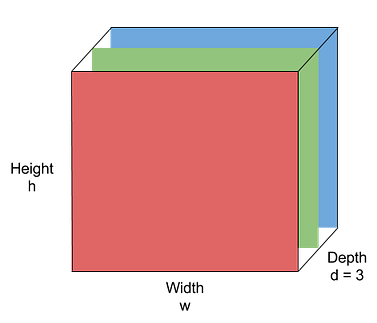Image by Purit Punyawiwat, Source : Datawow

## Main operations in CNN’s

Convolution operation

Convolution operation is (w.x+b) applied to all the different spatial localities in the input volume. Using more number of convolution operations helps to learn a particular shape even if its location in the image is changed.

Example: Generally clouds are present on the top of a landscape image. If an inverted image is fed into a CNN, more number of convolutional operations makes sure that the model identifies the cloud portion even if it is inverted.

Mathematical Expression: x_new = w.x + b where w is the filter/kernel, b is the bias and x is part of a hidden layer output. Both w and  _b _are different for every convolution operation applied on different hidden layers.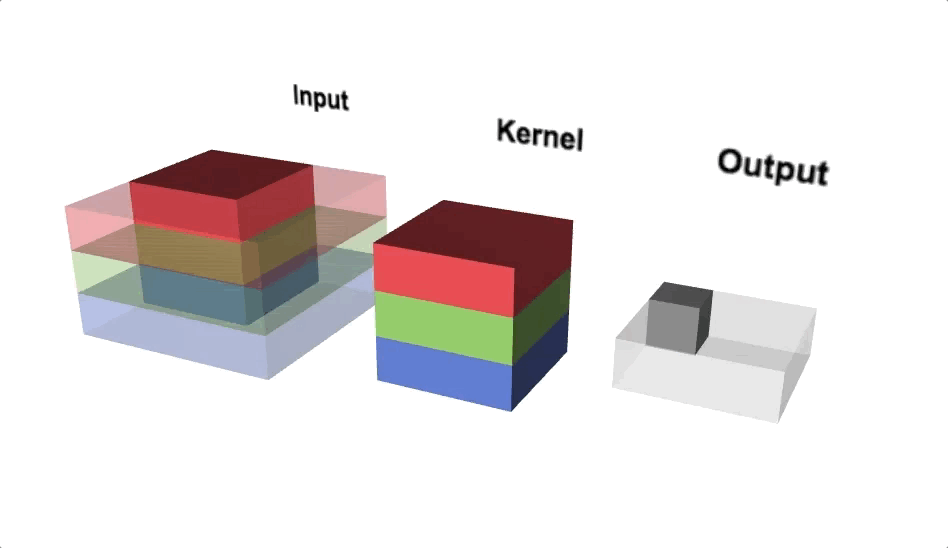Convolution Operations(Source: Lecture 22-EECS251, inst.eecs.berkley.edu)

Pooling

Pooling reduces the spatial dimensions of each activation map (output after convolution operation) while aggregating the localised spatial information. Pooling helps to squeeze the output from hidden layers along height and width. If we consider maximum value within the non-overlapping sub-regions then it is called Max-pooling. Max-pooling also adds non-linearity to the model.

#pytorch #deep-learning #transfer-learning #audio-classification #convolutional-network #neural networks1602983880

## Convolutional Neural Networks (CNN) Model from scratch for Object Detection

If you can’t explain it simply, you don’t understand it well enough - Einstein, the Man and His Achievement By G. J. Whitrow, Dover Press 1973.

CNN Model made from scratch, using the most popular Kaggle dataset  Fruits-360 and obtaining 98% accuracy.

Step 1- Importing Dataset From Kaggle to Google Colab

``````!pip install -q kaggle
``````
• Directly import the whole dataset to google colab and unzip the same
``````! mkdir ~/.kaggle
! cp kaggle.json ~/.kaggle/
! chmod 600 ~/.kaggle/kaggle.json

! mkdir fruits
! unzip fruits.zip -d fruits
``````

#image-recognition #cnn #data-science #convolutional-neural-net #neural-networks1600797360

## Fashion MNIST

Fashion Mnist is a Dataset created by Zolando Fashion Wear to replace the Original Mnist and at the same time increasing the difficulty.

This blog post is all about how to create a model to predict fashion mnist images and shows how to implement convolutional layers in the network

Let’s look at the code

#convolutional-neural-net #pytorch #convolutional-network #deep-learning1594274580

## CNN Series Part 1: How do computers see images?

In this article, we will learn about how computers see images & the issues faced while performing a computer vision task. We will see how deep learning comes into the picture & how with the power of neural networks, we can build a powerful computer vision system capable of solving extraordinary problems.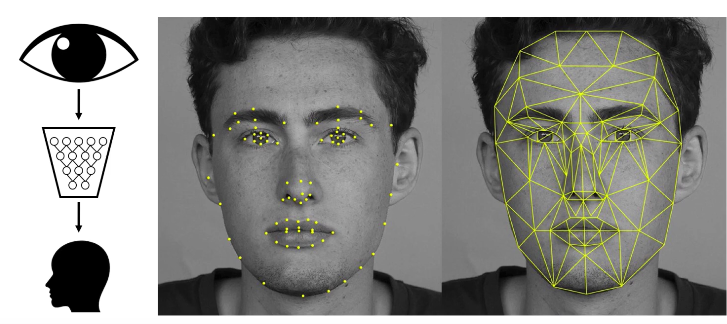One example of how deep learning is transforming computer vision is facial recognition or face detection. On the top left, you can see the icon of the human eye which visually represents vision coming into the deep neural network in the form of images, pixels, videos & on the output on the bottom you can see a depiction of the human face or detection of the human face or this could also be recognizing different human faces or emotions on the face and also the key facial features, etc.

#convolution-neural-net #computer-vision #neural-networks #cnn #convolutional-network #series1599214860

## Convolutional Neural Networks, Explained

A Convolutional Neural Network, also known as CNN or ConvNet, is a class of neural networks that specializes in processing data that has a grid-like topology, such as an image. A digital image is a binary representation of visual data. It contains a series of pixels arranged in a grid-like fashion that contains pixel values to denote how bright and what color each pixel should be.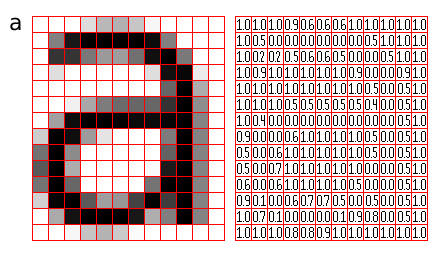Figure 1: Representation of image as a grid of pixels (Source)

The human brain processes a huge amount of information the second we see an image. Each neuron works in its own receptive field and is connected to other neurons in a way that they cover the entire visual field. Just as each neuron responds to stimuli only in the restricted region of the visual field called the receptive field in the biological vision system, each neuron in a CNN processes data only in its receptive field as well. The layers are arranged in such a way so that they detect simpler patterns first (lines, curves, etc.) and more complex patterns (faces, objects, etc.) further along. By using a CNN, one can enable sight to computers.

## Convolutional Neural Network Architecture

A CNN typically has three layers: a convolutional layer, a pooling layer, and a fully connected layer.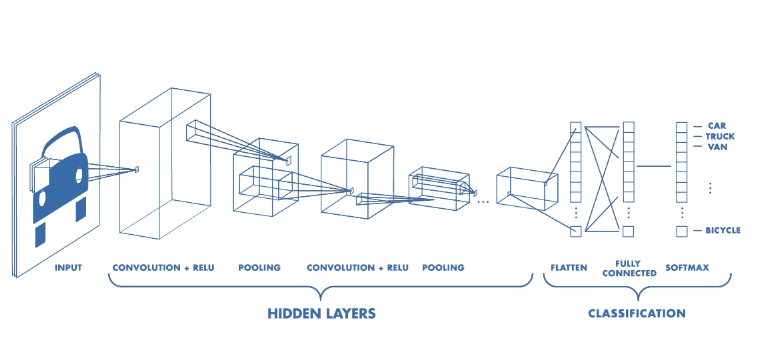Figure 2: Architecture of a CNN (Source)

## Convolution Layer

The convolution layer is the core building block of the CNN. It carries the main portion of the network’s computational load.

This layer performs a dot product between two matrices, where one matrix is the set of learnable parameters otherwise known as a kernel, and the other matrix is the restricted portion of the receptive field. The kernel is spatially smaller than an image but is more in-depth. This means that, if the image is composed of three (RGB) channels, the kernel height and width will be spatially small, but the depth extends up to all three channels.

#computer-vision #writing-nn #convolution-network #cnn #fashion-mnist #neural networks